Online Tution   »   CBSE Class 12 Mathematics Syllabus 2022-23

# CBSE Class 12 Mathematics Syllabus 2022-23

## CBSE Class 12 Mathematics Syllabus 2023

The Central Board of Secondary Education has recently released the CBSE Class 12 Mathematics Syllabus 2023 on its official website. We have provided the CBSE Class 12 Mathematics Syllabus 2023 in a detailed manner on this page to save the efforts of students. Mathematics requires practice, the more you practice the more you will be strong at this subject. The students must go through the entire CBSE Class 12 Mathematics Syllabus 2023 given on this page and bookmark this page to get all the latest updates from CBSE.

## Class 12 CBSE Mathematics Syllabus 2023

The CBSE Class 12 Mathematics Syllabus 2023 consists of six units. The units given in CBSE Class 12 Mathematics Syllabus 2023 are Relations and Functions, Algebra, Calculus, Vectors and Three-Dimensional Geometry, Linear Programming, and Probability. Check the marks distributed to each unit given in the table below:

 CBSE Class 12 Mathematics Syllabus 2023 Units No. of Periods Marks Unit 1:Relations and Functions 30 08 Unit 2: Algebra 50 10 Unit 3: Calculus 80 35 Unit 4: Vectors and Three-Dimensional Geometry 30 14 Unit 5: Linear Programming 20 05 Unit 6: Probability 30 08 Total 240 80 Internal Assessment 20

## Unit-Wise CBSE Class 12 Mathematics Syllabus 2023

#### CBSE Class 12 Mathematics Syllabus 2023: Unit 1

Unit 1: Relations and Functions

1. Relations and Functions: Types of relations: reflexive, symmetric, transitive and equivalence relations. One to one and onto functions.
2. Inverse Trigonometric Functions: Definition, range, domain, principal value branch. Graphs of inverse trigonometric functions.

#### CBSE Class 12 Mathematics Syllabus 2023: Unit 2

Unit 2: Algebra

1. Matrices: Concept, notation, order, equality, types of matrices, zero and identity matrix, transpose of a matrix, symmetric and skew-symmetric matrices. Operation on matrices: Addition and multiplication and multiplication with a scalar. Simple properties of addition, multiplication and scalar multiplication. Oncommutativity of multiplication of matrices and existence of non-zero matrices whose product is the zero matrix (restrict to square matrices of order 2). Invertible matrices and proof of the uniqueness of inverse, if it exists; (Here all matrices will have real entries).
2. Determinants: Determinants of a square matrix (up to 3 x 3 matrices), minors, co-factors and applications of determinants in finding the area of a triangle. Adjoint and inverse of a square matrix. Consistency, inconsistency and number of solutions of system of linear equations by examples, solving system of linear equations in two or three variables (having unique solution) using inverse of a matrix.

#### CBSE Class 12 Mathematics Syllabus 2023: Unit 3

Unit 3: Calculus

1. Continuity and Differentiability: Continuity and differentiability, chain rule, derivative of inverse trigonometric functions, ???? sin−1 ? , cos−1 ? and tan−1 ?, derivative of implicit functions. Concept of exponential and logarithmic functions. Derivatives of logarithmic and exponential functions. Logarithmic differentiation, derivative of functions expressed in parametric forms. Second order derivatives.
2. Applications of Derivatives: Applications of derivatives: rate of change of bodies, increasing/decreasing functions, maxima and minima (first derivative test motivated geometrically and second derivative test given as a provable tool). Simple problems (that illustrate basic principles and understanding of the subject as well as reallife situations).
3. Integrals: Integration as inverse process of differentiation. Integration of a variety of functions by substitution, by partial fractions and by parts, Evaluation of simple integrals of the following types and problems based on them.

dy/dx + py = q, where p and q are functions of x or constants.

d?/d? + px = q, where p and q are functions of y or constants.

#### CBSE Class 12 Mathematics Syllabus 2023: Unit 4

Unit 4: Vectors and Three-Dimensional Geometry

1. Vectors: Vectors and scalars, magnitude and direction of a vector. Direction cosines and direction ratios of a vector. Types of vectors (equal, unit, zero, parallel and collinear vectors), position vector of a point, negative of a vector, components of a vector, addition of vectors, multiplication of a vector by a scalar, position vector of a point dividing a line segment in a given ratio. Definition, Geometrical Interpretation, properties and application of scalar (dot) product of vectors, vector (cross) product of vectors.
2. Three-dimensional Geometry: Direction cosines and direction ratios of a line joining two points. Cartesian equation and vector equation of a line, skew lines, shortest distance between two lines. Angle between two lines.

#### CBSE Class 12 Mathematics Syllabus 2023: Unit 5

Unit 5: Linear Programming

1. Linear Programming: Introduction, related terminology such as constraints, objective function, optimization, graphical method of solution for problems in two variables, feasible and infeasible regions (bounded or unbounded), feasible and infeasible solutions, optimal feasible solutions (up to three non-trivial constraints).

#### CBSE Class 12 Mathematics Syllabus 2023: Unit 6

Unit 6: Probability

1. Probability: Conditional probability, multiplication theorem on probability, independent events, total probability, Bayes’ theorem, Random variable and its probability distribution, mean of a random variable.

## Question Paper Design Given in CBSE Class 12 Mathematics Syllabus 2023

 Typology of Questions Total Marks % Weightage Remembering: Exhibit memory of previously learned material by recalling facts, terms, basic concepts, and answers. Understanding: Demonstrate understanding of facts and ideas by organizing, comparing, translating, interpreting, giving descriptions, and stating main ideas 44 55 Applying: Solve problems to new situations by applying acquired knowledge, facts, techniques and rules in a different way. 20 25 Analysing: Examine and break information into parts by identifying motives or causes. Make inferences and find evidence to support generalizations Evaluating: Present and defend opinions by making judgments about information, validity of ideas, or quality of work based on a set of criteria. Creating: Compile information together in a different way by combining elements in a new pattern or proposing alternative solutions 16 20 Total 80 100
1. No chapter wise weightage. Care to be taken to cover all the chapters
2. Suitable internal variations may be made for generating various templates keeping the overall weightage to different form of questions and typology of questions same.

Choice(s): There will be no overall choice in the question paper. However, 33% internal choices will be given in all the sections

## CBSE Class 12 Mathematics Syllabus 2023: Internal Assessment

The internal assessment of Mathematics consists of 20 marks. Internal assessment of mathematics is divided into two parts. Check the details and mark the distribution of both the parts given below in the table:

 Internal Assessment Marks Periodic Tests ( Best 2 out of 3 tests conducted) 10 Mathematics Activities 10 Total 20

Conduct of Periodic Tests: Periodic Test is a Pen and Paper assessment which is to be conducted by the respective subject teacher. The format of the periodic test must have questions items with a balanced mix, such as, very short answer (VSA), short answer (SA) and long answer (LA) to effectively assess the knowledge, understanding, application, skills, analysis, evaluation and synthesis. Depending on the nature of subject, the subject teacher will have the liberty of incorporating any other types of questions too. The modalities of the PT are as follows:
a) Mode: The periodic test is to be taken in the form of pen-paper test.
b) Schedule: In the entire Academic Year, three Periodic Tests in each subject may be conducted as follows:

 Test Pre Mid-term (PT-I) Mid-Term (PT-II) Post Mid-Term (PT-III) Tentative Month July-August November December-January

This is only a suggestive schedule and schools may conduct periodic tests as per their convenience. The winter bound schools would develop their own schedule with similar time gaps between two consecutive tests.

c) Average of Marks: Once schools complete the conduct of all the three periodic tests, they will convert the weightage of each of the three tests into ten marks each for identifying best two tests. The best two will be taken into consideration and the average of the two shall be taken as the final marks for PT.

d) The school will ensure simple documentation to keep a record of performance as suggested in detail circular no.Acad-05/2017.

e) Sharing of Feedback/Performance: The students’ achievement in each test must be shared with the students and their parents to give them an overview of the level of learning that has taken place during different periods. Feedback will help parents formulate interventions (conducive ambience, support materials, motivation and morale-boosting) to further enhance learning. A teacher, while sharing the feedback with student or parent, should be empathetic, non- judgmental and motivating. It is recommended that the teacher share best examples/performances of IA with the class to motivate all learners.

## Prescribed Books for CBSE Class 12 Mathematics Syllabus 2023

2) Mathematics Part I – Textbook for Class XII, NCERT Publication
3) Mathematics Part II – Textbook for Class XII, NCERT Publication

Related post:

## FAQs on CBSE Class 12 Mathematics Syllabus 2023

Q. Will CBSE conduct CBSE Class 12 Exam 2023 annually?
Yes, CBSE has recently announced that from the next session CBSE will conduct annual board exams.
Q. Where I can get CBSE Class 12 Mathematics Syllabus 2023?
You can get CBSE Class 12 Mathematics Syllabus 2023 from this page. To get the syllabus of other subjects the students can visit the official website of Adda247 School.
Q. How many units are there in CBSE Class 12 Mathematics Syllabus 2023?
CBSE Class 12 Mathematics Syllabus 2023 consists of 5 units. The units of CBSE Class 12 Mathematics Syllabus 2023 are Relations and Functions, Algebra, Calculus, Vectors and Three-Dimensional Geometry, Linear Programming, and Probability.

Sharing is caring!

## FAQs

### Will CBSE conduct CBSE Class 12 Exam 2023 annually?

Yes, CBSE has recently announced that from the next session CBSE will conduct annual board exams.

### Where I can get CBSE Class 12 Mathematics Syllabus 2023?

You can get CBSE Class 12 Mathematics Syllabus 2023 from this page. To get the syllabus of other subjects the students can visit the official website of Adda247 School.

### How many units are there in CBSE Class 12 Mathematics Syllabus 2023?

CBSE Class 12 Mathematics Syllabus 2023 consists of 5 units. The units of CBSE Class 12 Mathematics Syllabus 2023 are Relations and Functions, Algebra, Calculus, Vectors and Three-Dimensional Geometry, Linear Programming, and Probability.

### TOPICS:

•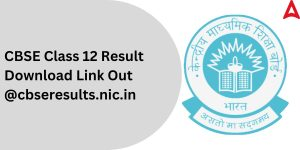CBSE 12th Result 2023 Out, Download link...
•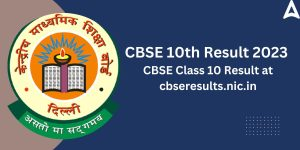CBSE 10th Result 2023 Out, Check CBSE Cl...
•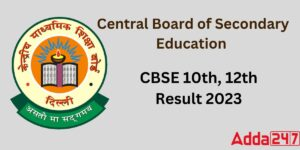CBSE Result 2023 Out, Check Class 12th B...
•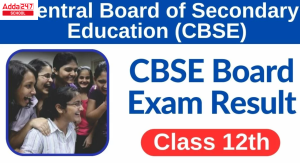CBSE Class 12th Result 2023 Out, Check @...
•CBSE 10th Result 2023 Out, Download Link...
•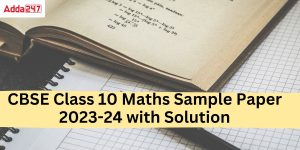Class 10 Maths Sample Paper 2023-24 with...